## Homework Problems

1. For the two vectors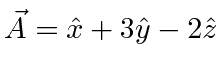and, find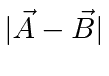, the component of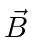along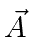, the angle betweenand,, and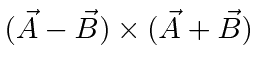.

2. The gradient operator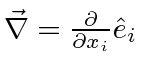transforms like a vector. Use this to write the equations of electromagnetism with the Einstein summation convention, that is, with no vector symbols. To make it interesting, here are the EM equations in Rationalized Heaviside-Lorentz units.As an example, we can write the first equation.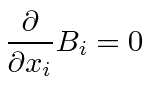3. Use the operatorto show that the divergence of a curl is zero.Also calculate the curl of the curl.4. Use the totally antisummetric tensor to derive the identity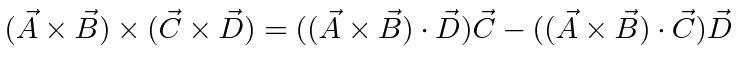.

5. Calculate the rotation matrix for a passive rotation about theaxis through an anglefollowed by a rotation about the new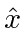axis by an angle.

6. Calculate the rotation matrix for a passive rotation through an angle of 120 degrees about an axis making equal angles with the original three coordinte axes.

7. Calculate the time derivative of a rotation matrix through an angleabout theaxis. Remembering that the axial vectoris related to an antisymmetric tensor, relate the time derivative of the rotation matrix (evaluated at) to.

8. Show that for matrices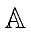and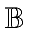,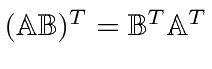, and that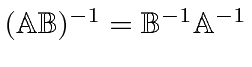.

Jim Branson 2012-10-21